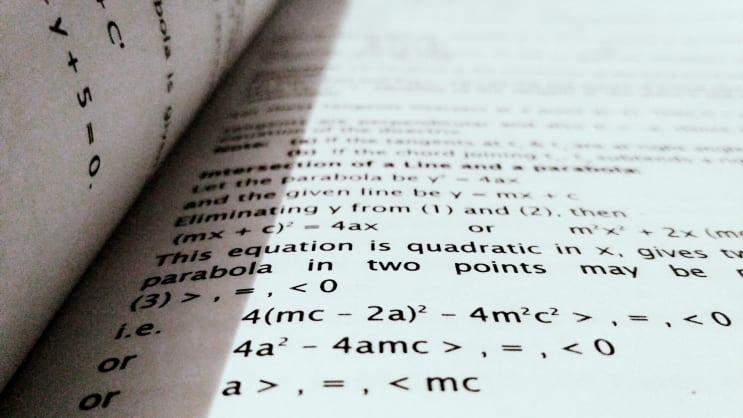# Bachelor in Mathematical and Computer Modelling

### Program Description

The program includes courses that allow getting acquainted with the modern and classical directions of mathematics and computer modeling. Our graduates gain sufficient knowledge to conduct basic research in such areas as algebra, geometry, differential equations, and differential equations with partial derivatives. The knowledge gained by them can be useful for work in the oil and gas field (calculations and construction of models of hydrodynamic processes), financial branches, and the IT industry. In the course of our program, students gain the skills of high-performance computing and simulation of various processes, for example, the problems of transport streams, optimizing tasks, etc.#### Content of an educational program

• Calculus I – The concept of numerical function. Elementary functions and their graphs. Main properties. Limit and continuity of function. Derivative and its applications.
• Calculus II – The antiderivative. Definite integral. Applications of definite integrals. The simplest differential equations of the first order.
• Linear algebra – methods of solving the system of linear algebraic equations. Matrixes and actions over them. Determinants. Elements of vector algebra. Linear geometrical objects (a straight line on the plane and in space, the plane). Curves and surfaces of the second order. Linear operators. Eigen-values and eigenvectors of a linear operator. Quadratic forms and reduction of quadratic forms to a canonical form.
• Calculus of probabilities and mathematical statistics, Mathematics IV - Accidental events, and operations over them. Classical and geometrical determination of probability. Basic formulas of probability calculation. Bernoulli's scheme. Discrete random variables. Continuous random variables. Elements of mathematical statistics.
• Numerical methods, Mathematics III – The basics of work with the systems of analytical and numerical calculations MATLAB are studied in this course. The basics of the error theory. Numerical solution of nonlinear equations. Numerical solution of the systems of linear equations. Interpolation. Numerical differentiation. Numerical integration. Numerical solution of differential equations.
• Mathematics I – The elements of linear algebra. The elements of analytical geometry. Introduction to analysis. Differential calculus of function of one variable. The functions of several variables.
• Mathematics II – Integral calculus. Complex numbers. Differential equations. Multiple Integrals. Series. The elements of field theory.
• Ordinary differential equations - Differential equations of the first order. Linear differential equations with constant coefficients. The systems of differential equations. Elementary boundary-value problems (heat conduction equation, wave equation, Laplace's equation).
• Optimization and Control Circuit - Linear programming. Project Management. Dynamic programming. Integer programming. Nonlinear programming. Games theory.

Contact details:

Address: Almaty, Tole Bi St., 59, 332 cab.
Phone: +7 (727) 2725615, 2725129
Email: study.international@kbtu.kz

Last updated Nov 2020

KBTU considers its mission to provide high-quality education for future leaders in business and industry, to promote innovative technologies and research in the most advanced and prospective areas of ... Read More

KBTU considers its mission to provide high-quality education for future leaders in business and industry, to promote innovative technologies and research in the most advanced and prospective areas of science for the benefit of the region and the country as a whole. The strategic vision of the University is to promote the ideals of academic excellence, scientific progress, and national well-being, to maintain the status of an undisputed leader in the field of technical and business education. The major goals and ambitions of the university are the creation of a scientific and educational center with highly qualified scientific and pedagogical potential and a developed modern material and technical base, the development of a dual vocational training, training of highly qualified specialists who have professional and personal competencies with emphasis on financial, power-generating sector, spheres of high and information technologies and the maritime industry. Read less
Almaty